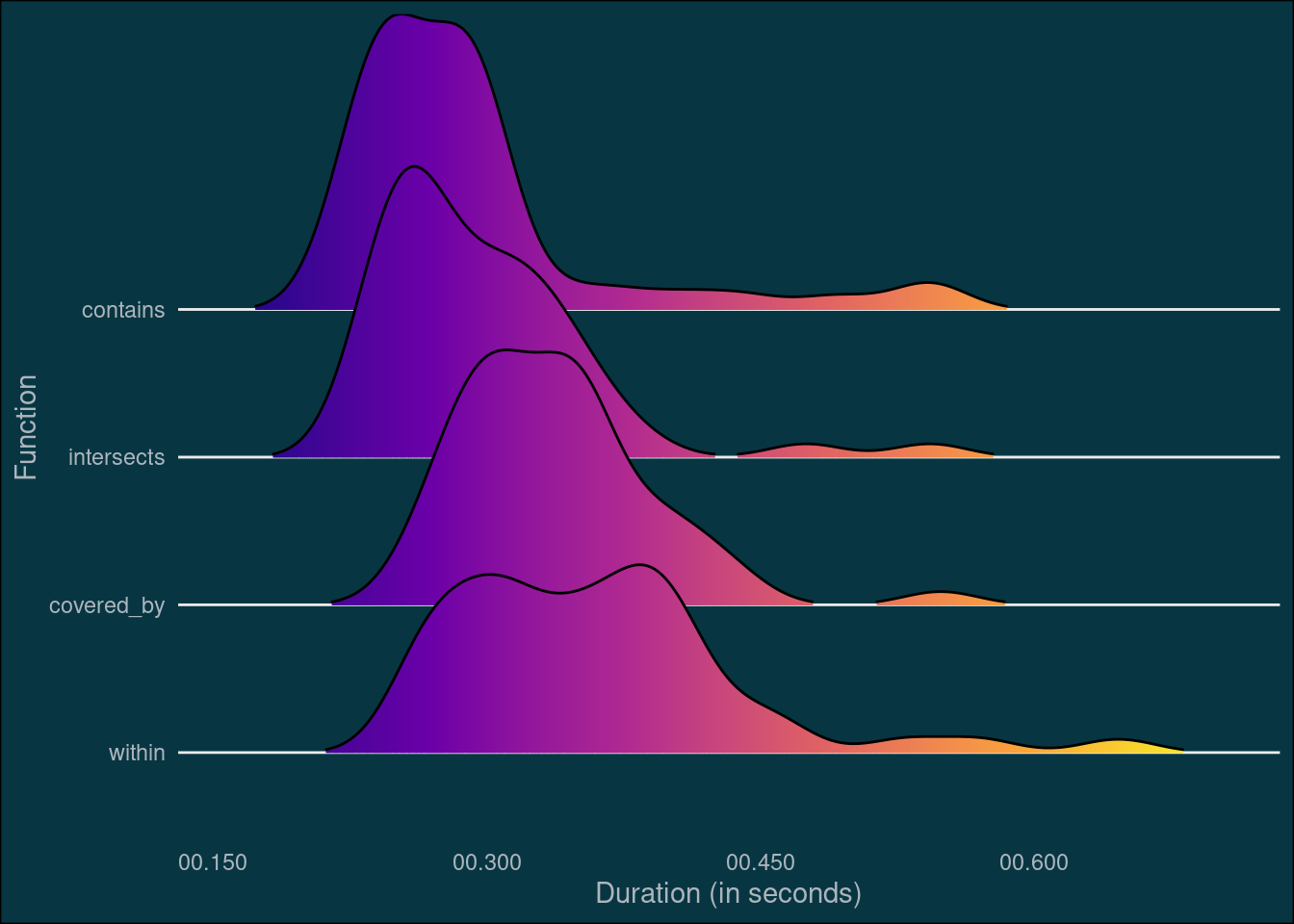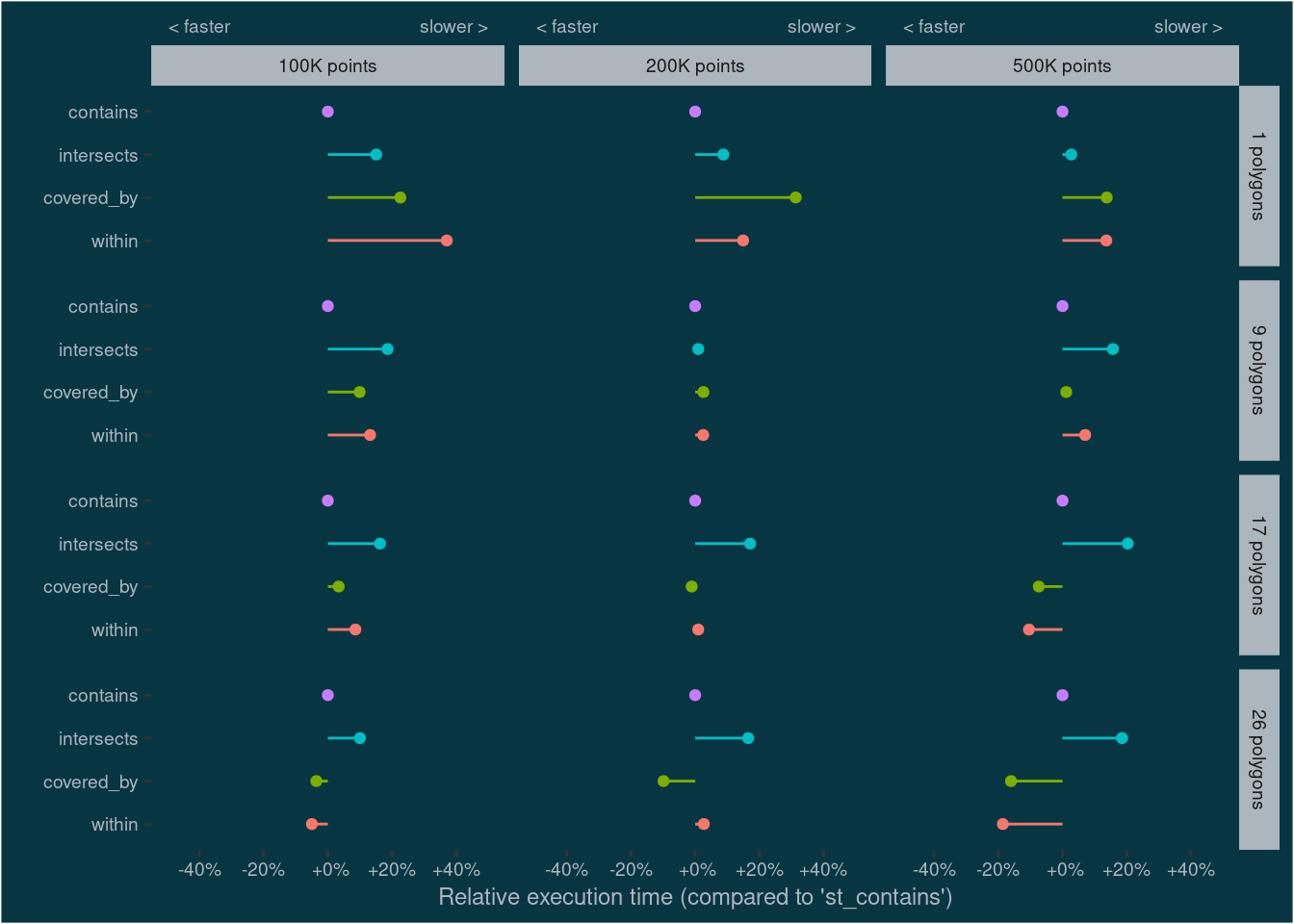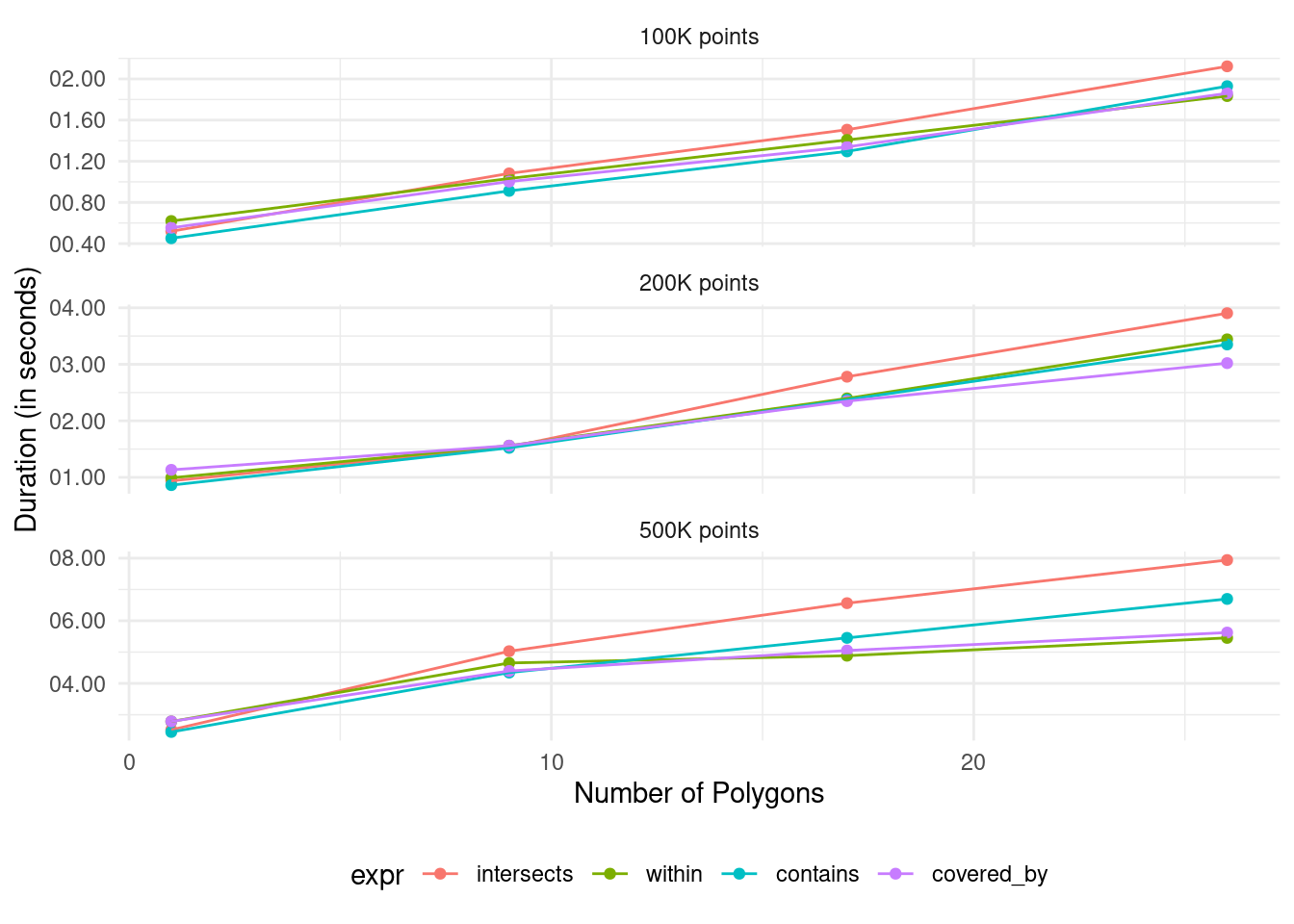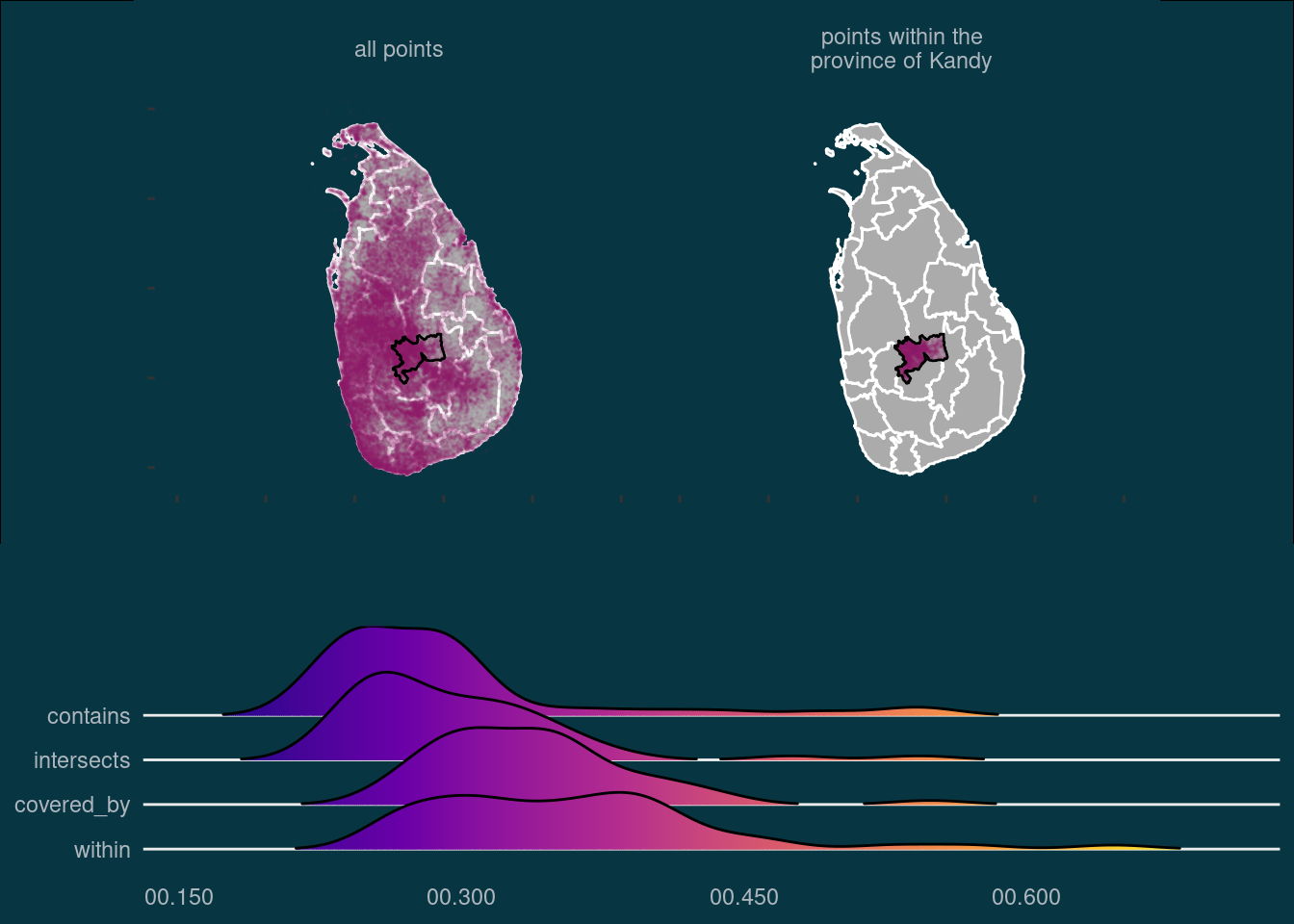# Benchmarking binary predicates

Comparing the performance of different methods to do a “point in polygon operation” with sf.

Author

Nils Ratnaweera

Published

October 22, 2021

I often work with geodata in `R` and come across situations where I need to subset points based on whether they lie within a polygon or not. There are several functions to solve this problem1. From the package `sf`, the functions `st_within`, `st_contains`, `st_intersects` and `st_covered_by` can all answer this question2. I noticed that with big datasets, some of these functions are unbearably slow. To find out which one is faster in which scenario, I decided to benchmark these four functions.

To make things more interesting, I won’t use my usual Swiss data for this test, but data from my second home, Sri Lanka. More specifically: I will use the Geonames data (> 50k points) and the administrative boundaries of Sri Lanka (26 polygons).

``````library(dplyr)
library(purrr)
library(sf)
library(ggplot2)
library(lubridate)
library(microbenchmark)
library(ggridges)
library(forcats)``````
preparing boundary data
``````# Downloaded from: https://data.humdata.org/dataset/sri-lanka-administrative-levels-0-4-boundaries
# Administrative Level 0: country (1 features)
# Administrative Level 1: province (9 features)
# Administrative Level 2: district (26 features)
# Administrative Level 3: divisional secretatiat (333 features)

tmp <- tempdir()

boundary_dir <- file.path(tmp, "boundary")

)
# https://epsg.io/5234
# https://epsg.io/5235``````
preparing geonames datase
``````# geonameid         : integer id of record in geonames database
# name              : name of geographical point (utf8) varchar(200)
# asciiname         : name of geographical point in plain ascii characters, varchar(200)
# alternatenames    : alternatenames, comma separated, ascii names automatically transliterated, convenience attribute from alternatename table, varchar(10000)
# latitude          : latitude in decimal degrees (wgs84)
# longitude         : longitude in decimal degrees (wgs84)
# feature class     : see http://www.geonames.org/export/codes.html, char(1)
# feature code      : see http://www.geonames.org/export/codes.html, varchar(10)
# country code      : ISO-3166 2-letter country code, 2 characters
# cc2               : alternate country codes, comma separated, ISO-3166 2-letter country code, 200 characters
# admin1 code       : fipscode (subject to change to iso code), see exceptions below, see file admin1Codes.txt for display names of this code; varchar(20)
# admin2 code       : code for the second administrative division, a county in the US, see file admin2Codes.txt; varchar(80)
# population        : bigint (8 byte int)
# elevation         : in meters, integer
# dem               : digital elevation model, srtm3 or gtopo30, average elevation of 3''x3'' (ca 90mx90m) or 30''x30'' (ca 900mx900m) area in meters, integer. srtm processed by cgiar/ciat.
# timezone          : the iana timezone id (see file timeZone.txt) varchar(40)
# modification date : date of last modification in yyyy-MM-dd format

colnames <- c("geonameid", "name",  "asciiname",  "alternatenames", "latitude",
"longitude",  "feature_class",  "feature_code", "country_code",
"modification_date")

geonames_dir <- file.path(tmp, "geonames")

unzip("data-git-lfs/LK.zip", exdir = geonames_dir)

geonames <- read_tsv(file.path(geonames_dir, "LK.txt"),col_names = colnames) %>%
st_as_sf(coords = c("longitude", "latitude"), crs = 4326)``````
``````Rows: 56748 Columns: 19
── Column specification ────────────────────────────────────────────────────────
Delimiter: "\t"
chr   (8): name, asciiname, alternatenames, feature_class, feature_code, cou...
date  (1): modification_date

ℹ Use `spec()` to retrieve the full column specification for this data.
ℹ Specify the column types or set `show_col_types = FALSE` to quiet this message.``````

Once all the data is imported, I can demonstrate visually the task. I want to subset all points within the province of Kandy (incidentally where I spent 5 superb years of my childhood). Using `st_within()` for this operation, the output looks like this:

subsetting and creating a map
``````kandy <- filter(sl_boundary_l2, ADM2_EN == "Kandy")

points_filter <- list(
within = geonames[st_within(geonames,kandy,sparse = FALSE)[,1],]
)

plot_bg_col <- Sys.getenv("plot_bg_col")
text_col <- Sys.getenv("text_col")

p1 <- ggplot(sl_boundary_l2) +
geom_sf(color = "#ffffff", fill = "#ababab") +
geom_sf(data = rbind(transmute(geonames, val = "all points"),
transmute(points_filter[["within"]],
val = "points within the\nprovince of Kandy")),
alpha = 0.05, size = 0.05, color = "#8d2663") +
geom_sf(data = ~filter(., ADM2_EN == "Kandy"), fill = NA, color = "#000000") +
facet_wrap(~val) +
coord_sf(xlim = c(78, 83)) +
theme(strip.background = element_blank(),
strip.text = element_text(color = text_col),
panel.background = element_blank(),
plot.background = element_rect(fill = plot_bg_col),
panel.grid = element_blank(),
axis.text = element_blank(),
)

p1``````Next, I will do the same operation with the other functions and also check the output number of rows to see if they are similar (they might be slightly off if we have points exactly on the polygon boundary) or even identical.

``````points_filter[["contains"]] <- geonames[st_contains(kandy,
geonames,
sparse = FALSE)[1,],]
points_filter[["intersects"]] <- geonames[st_intersects(geonames,
kandy,
sparse = FALSE)[,1],]
points_filter[["covered_by"]] <- geonames[st_covered_by(geonames,
kandy,
sparse = FALSE)[,1],]

tibble(
function_name = names(points_filter),
nrow = sapply(points_filter, nrow),
identical_to_st_within = sapply(points_filter, function(x){
identical(points_filter[["within"]], x)
})
) %>%
knitr::kable(col.names = stringr::str_replace_all(colnames(.),"_", " "))``````
function name nrow identical to st within
within 3251 TRUE
contains 3251 TRUE
intersects 3251 TRUE
covered_by 3251 TRUE

To find out which function is the fastest, I use the package `microbenchmark`. Since it doesn’t always take the same amount of time to process the same function, each function is executed multiple times (`times = 50`) and we will look at the distribution of the execution times.

Benchmarking the functions
``````mbm  <- microbenchmark(
intersects = st_intersects(kandy,geonames),
within = st_within(geonames,kandy),
contains = st_contains(kandy,geonames),
covered_by = st_covered_by(geonames,kandy),
times = 50
)``````
visualizing the result
``````mbm2df <- function(mbm_obj){
df <- as.data.frame(mbm_obj)
df\$time <- dnanoseconds(df\$time)
df
}

mbm_df <- mbm2df(mbm)

p2 <- mbm_df %>%
mutate(
expr = fct_reorder(expr,time,median,.desc = TRUE)
) %>%
ggplot(aes(time,expr,fill = ..x..)) +
geom_density_ridges_gradient(scale = 2, rel_min_height = 0.01) +
scale_fill_viridis_c(option = "C")  +
scale_x_time(name = "Duration (in seconds)",
labels = scales::time_format(format = "%OS3")) +
labs(y = "Function") +
theme_minimal() +
theme(legend.position="none",
plot.background = element_rect(fill = plot_bg_col), panel.grid.minor.x  = element_blank(),panel.grid.major.x = element_blank(),  axis.text = element_text(colour = text_col), text = element_text(colour = text_col))

p2``````
``Picking joint bandwidth of 0.0204``This benchmark shows that `st_contains` and `st_intersects` executes faster than `st_covered_by` and `st_within`. The next question is: How do the functions scale and perform under different scenarios? I’ll test this by generating additional points to subset, and also by using more provinces than just Kandy. And since I’m more interested in relative rather than absolute execution times, I will calculate the median duration per function and scenario and rescale the values by deviding them with the duration of `st_intersects`.

Benchmarking scalability
``````n_points_vec <- c(100e3,200e3,500e3)
n_poly_vec <- c(1,9,17,26)

mbm2 <- map_dfr(n_points_vec,function(n_points){

points <- st_sample(sl_boundary_l2,n_points,what = "centers")

mbm_points <- map_dfr(n_poly_vec, function(n_poly){

polygons <- sample_n(sl_boundary_l2, n_poly)

mbm_poly <- microbenchmark(
intersects = st_intersects(polygons,points),
within = st_within(points,polygons),
contains = st_contains(polygons,points),
covered_by = st_covered_by(points,polygons),
times = 10
)

as_tibble(mbm_poly) %>%
mutate(n_poly = n_poly)
}) %>%
mutate(n_points = n_points)
})``````
Visualizing results
``````mbm2_df <- mbm2df(mbm2)

mylabels <- function(x){sprintf("%+3.f%%", x*100)}

mbm2_df %>%
group_by(expr, n_poly, n_points) %>%
summarise(median = median(time)) %>%
ungroup() %>%
group_by(n_poly,n_points) %>%
mutate(
perc = median/median[expr == "contains"]-1,
expr = fct_relevel(expr, "within", "covered_by","intersects", "contains")
) %>%
ggplot(aes(perc,as.factor(expr), color = expr, fill = expr)) +
geom_point() +
geom_linerange(aes(xmin = 0, xmax = perc)) +
# expand_limits(x = 0) +
scale_x_continuous("Relative execution time (compared to 'st_contains')",
breaks = seq(-.4,.4,.2), labels = mylabels,
limits = c(-.5,.5),
sec.axis = sec_axis(~.x,
breaks = c(-.4,.4),
labels = c("< faster","slower > "))) +
labs(y = "") +
facet_grid(n_poly~n_points,
labeller = labeller(n_points = ~paste0(as.integer(.x)/1e3, "K points"),
n_poly = ~paste0(.x, " polygons")))+
# theme_light() +
theme(legend.position = "none",
axis.ticks.x.top = element_blank(),
text = element_text(size = 9),
plot.background = element_rect(fill = plot_bg_col),
panel.background = element_rect(fill = plot_bg_col),
panel.grid = element_blank(),axis.text = element_text(colour = text_col),axis.title = element_text(colour = text_col),strip.background = element_rect(fill = text_col)
)``````visualizing the result
``````mbm2_df %>%
group_by(expr, n_poly, n_points) %>%
summarise(median = median(time)) %>%
ungroup() %>%
ggplot(aes(n_poly,median, color = expr, fill = expr)) +
geom_point() +
geom_line()  +
scale_y_time(name = "Duration (in seconds)",
labels = scales::time_format(format = "%OS2")) +
scale_x_continuous(name = "Number of Polygons") +
facet_wrap(~n_points,
labeller = labeller(n_points = ~paste0(as.integer(.x)/1e3, "K points")),
scales = "free_y", ncol = 1) +
theme_minimal() +
theme(legend.position = "bottom")``````This test shows something interesting: While `st_contains` and `st_intersects` are fast with a single polygon, they don’t scale well with lager number of polygons. This effect is especially prominent when the number of points is large (~500K).

My take home message from this whole exercise: If you want to subset points based on whether they lie in specific polygons or not, use `st_intersects` or `st_contains` when the number of polygons is small. Use `st_covered_by` or `st_within` when the number of polygons is large. If it’s important what happens to points lying on the edge, remember that only `st_intersects` and `st_covered_by` will include them.## Footnotes

1. especially since I mostly don’t care what happens to point which lie exactly on the polygon edge↩︎

2. `st_within` and `st_contains` will disregard points on a line, `st_intersects` and `st_covered_by` will include them↩︎